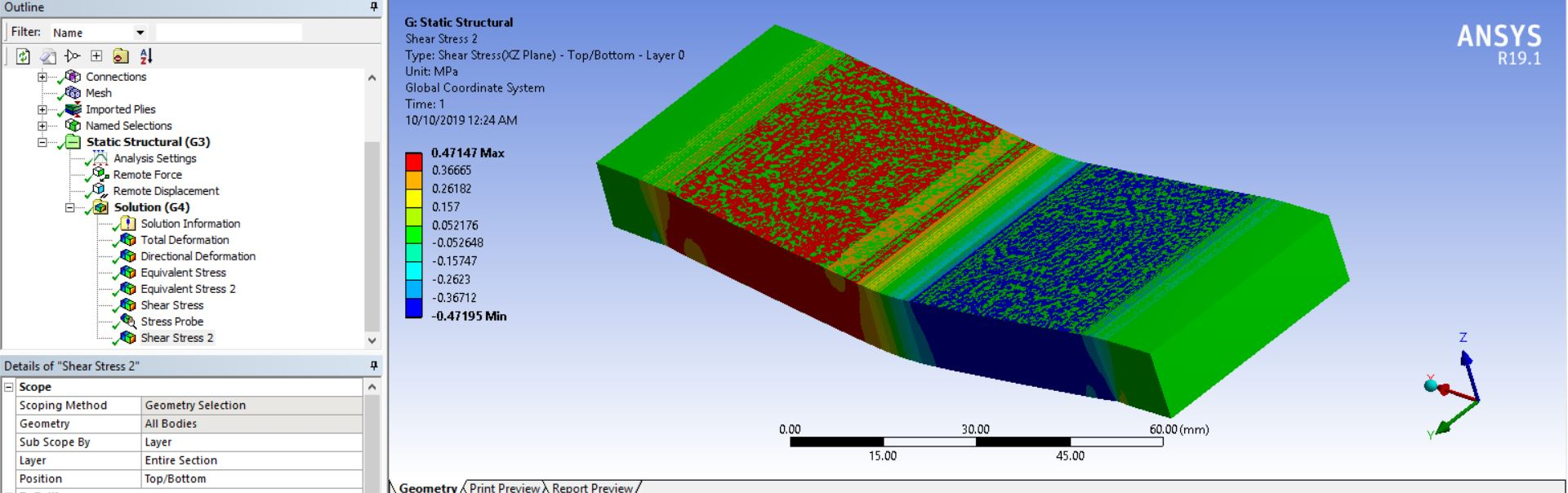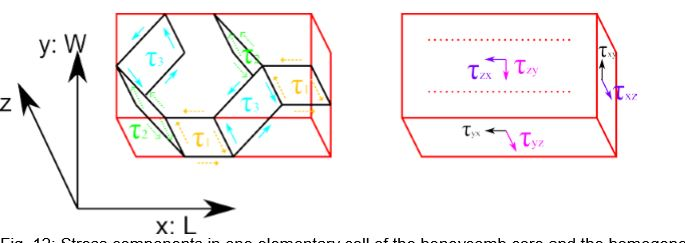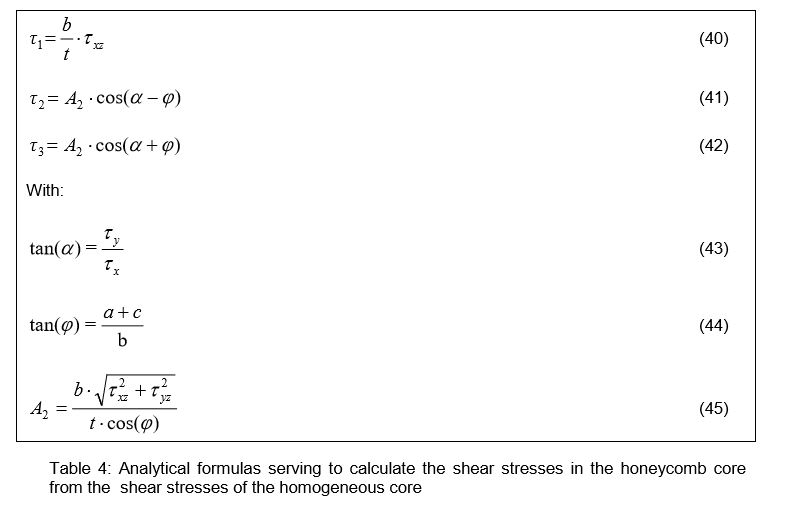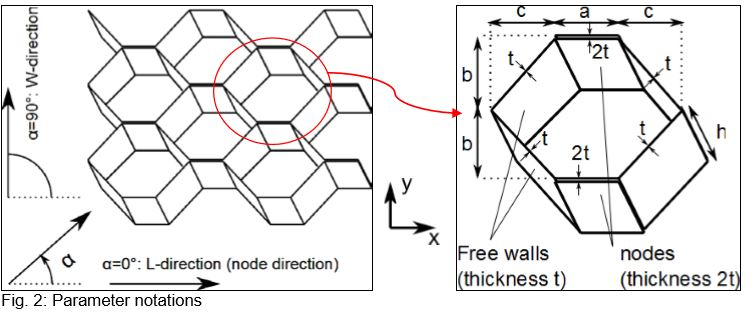# shear stresses in the homogenized core of sandwich panel

I am trying to simulate the 3 point bending test of sandwich composite. For this i replaced the honeycomb core geometry by solid core after giving the same orthotropic properties to the solid core.(homogenized)

The bending stress in the face sheet and the central deflection of the sandwich matches the analytical result.

The value of shear stress in the honeycomb core by analytical method is 0.369 Mpa. While the shear stress in the solid core is about 0.471 Mpa.

My questions are:

1. This method holds good for the deflection and bending stress in the face sheet but can i expect the similar accuracy in the stresses in solid core.

2.The below research paper suggests the relation between the shear stresses in the solid core geometry to the honeycomb geometry. But when i put the values to check it , the difference between the analytical and FEA values increases by a great margin.

LITERATURE

ANALYTICAL METHOD TO FIND OUT SHEAR STRESS IN HONEYCOMB CORE:-

shear stress in xz plane = P/(D+C)xB

Where P is the load = 400

C is core height = 12.7 mm

Dis sandwich height=13.1 mm

b is sandwich width=42 mm

so value comes out to be = 0.369 MPa

ANSYS:-

Shear stress in the xz plane is 0.471 MpaLITERATURE 2

Another research paper established a relationship between the shear stresses in the actual honeycomb core and homogenized solid core.b = 5.499mm , t =0.2 mm

so as per the first relation

shear stress in the honeycomb core = (b/t)x shear stress in XZ plane of solid core.

b/t = 27.496

But

shear stress in honeycomb core =0.369Mpa

shear stress in homoginesed core ( by ansys) =0.471 Mpa

so the above relation is not satisfied.

here is the research paper.